Code-Caffe

AffordanceNet， 主要用于从 RGB 图片中同时检测多种 objects 以及其 affordance.

• deconvolutional layers
• 鲁棒的 resizing 策略
• multi-task loss 函数

## 1. 问题阐述

• object 位置：相对于图片左上角的方框位置；
• object 类别：方框内 object 类别；
• object affordance：方框内每一个像素的 object 类别. 具有相同功能的 object 像素区域被作为一个 affordance.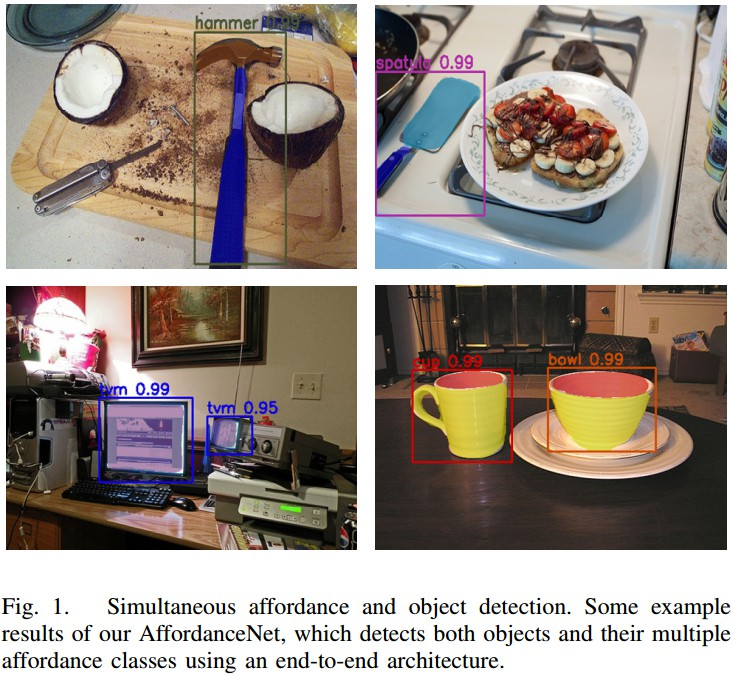## 2. AffordanceNet 网络结构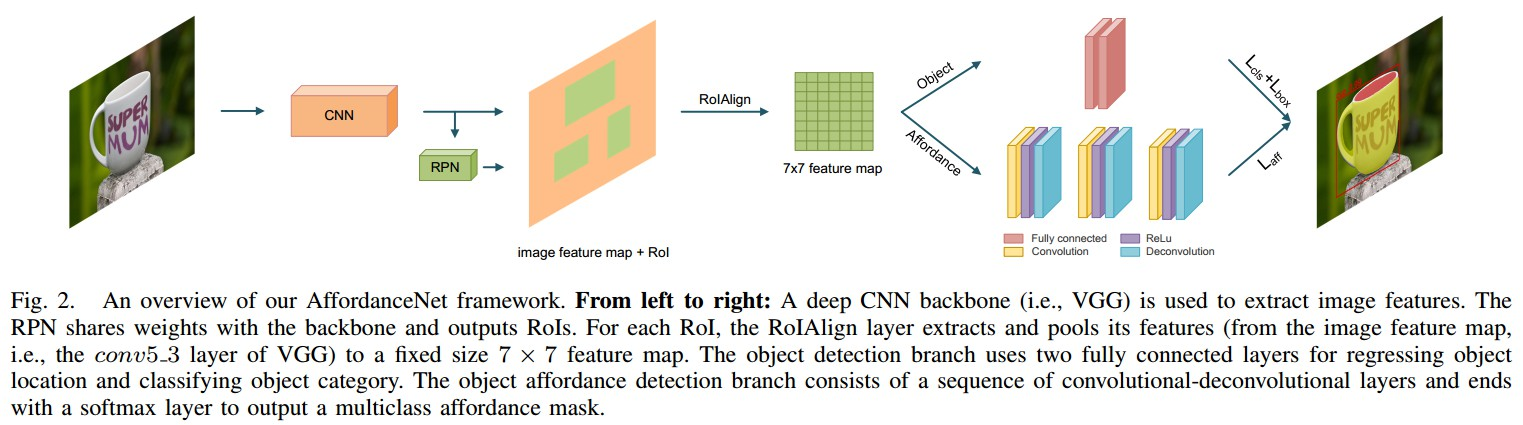• CNN backbone - 如VGG，用于提取图片特征；
• RPN - 与 CNN backbone 共享权重参数，并输出 RoIs；
• RoI alignment(RoIAlign) 层- Mask R-CNN中的 RoI 对齐层，对于每个 RoI，RoIAlign 从图片 feature map(如，VGG 的 conv5_3 层的输出) 中提取和池化特征，得到固定尺寸 7×7 的 feature map.
• object 检测网络分支 - 采用两个全连接层来回归 object 位置，并分类；
• object affordance 检测分支 - 包括一序列的 conv-deconv 层，以得到平滑精确的 affordance map；其最后一层接 softmax 层以输出 multiclass affordance mask.

### 2.1 RoIAlign

Faster R-CNN-RPN，RPN 与 backbone 网络共享权重，并输出在不同 size 的 bounding boxes(RoI/object proposal)；

RoI Pool 层通过将每个 RoI 划分为规则网格，再采用 max-pooling 对每个网格单元的 feature map values 进行处理.

RoIAlign 对于像素级任务，如图像分割，十分重要.

### 2.2 高分辨率 Affordance Mask 的 Deconvolution

Mask R-CNN 和 FCIS 等实例分割方法，采用固定尺寸 mask (如，14×14，28×28) 来表示 object 的 segmentation mask. 对每个 RoI 预测的 mask 的像素值都是二值，前景或背景，是可行的.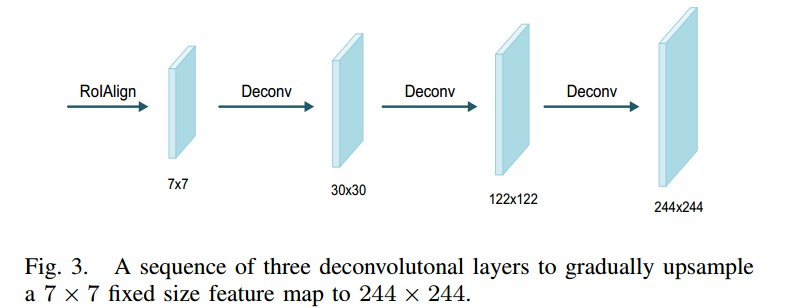${ S_o = s * (S_i- 1) + S_f- 2*d }$

RoIAlign 层输出的 feature map 尺寸是 7×7. 如图 Fig 3，采用三个 deconv 层可以上采样到更高的分辨率 244 × 244.

• deconv1 - d=1, s=4, S_f = 8
• deconv2 - d=1, s=4, S_f = 8
• deconv3 - d=1, s=2, S_f = 4

### 2.3 Robust Resizing Affordance Mask

${ \hat{p} }$ 是 ${ \hat{P} }$ 中的值 ；
${ \alpha }$ 是超参数，这里设为 0.005.

### 2.4 End-to-End 网络结构

RPN 与 backbone 网络共享权重参数，并输出候选 bounding boxes(RoIs)；

object 检测分支，采用两个全连接层，其输出均为 4096，其后接 classification 层和回归层，对 object 进行分类并回归 object 位置；

affordance 检测分支，将 7×7 的feature map 多重 deconv 上采样到 244 ×244 的分辨率；然后，采用 softmax 层对 244×244 map 内的每个像素划分到最可能的 affordance 类别.

## 3. Mulit-task Loss

classification 分类层输出 K+1 个 object 类别的概率分布 ${ p = (p_0,...,p_K) }$；

regression 回归层输出 K+1 个 bounding boxes 偏移值，每个偏移值包括 box center 和 box size：${ t^k = (t_x^k, t_y^k,t_w^k,t_h^k) }$. 每一个偏移值 ${ t^k }$ 对应一个类别 k.

affordance 检测分支输出RoI内每个像素的概率分布集合 ${ m = \lbrace m^i \rbrace_{i \in RoI} }$，其中，${ m^i = (m_0^i, ...,m_C^i) }$，是关于 C+1 个 affordance labels，softmax 层的输出. 包括 background.

Multi-task Loss:

${ L = L_{cls} + L_{loc} + L_{aff} }$

${ L_{cls} }$ - 分类层的输出
${ L_{loc} }$ - 回归层的输出
${ L_{aff} }$ - affordance 检测的输出

${ u }$ 和 ${ v }$ 是训练数据集提供的，mask ${ s }$ 是 RoI 和其对应的 groundtruth mask 的交集. 将不属于 RoI 中不属于该交集的像素，标记为 background.

target mask 被 resized 到固定尺寸，如 244 × 244，因此 Loss 可以转化为：

${ L(p, u, t^u, v, m, s) = L_{cls}(p, u) + I[u \geq 1] L_{loc}(t^u, v) + I[u \geq 1] L_{aff}(m, s) }$

${ L_{cls}(p,u) }$ - 分类任务，多项式交叉熵损失函数(multinomial cross entropy loss)：

${ L_{cls}(p, u) = -log(p_u) }$

${ L_{loc}(t^u, v) }$ - 回归任务，Smooth L1 loss，计算回归的 box offset ${ t^u }$(对应于groundtruth object 类别 ${ u }$) 与 groundtruth box offset ${ v }$ 间的 smooth l1 loss 值：

${ L_{loc}(t^u, v) = \sum_{i \in {x, y, w, h}} Smooth_{L1}(t_i^u - v_i) }$

${ L_{aff}(m, s) }$ - affordance 检测，多项式交叉熵损失函数：

${ L_{aff} = \frac{-1}{N} \sum {i \in RoI} log(m {s_i}^i) }$

${ I[u \geq 1] }$ - 如果 ${ u \geq 1 }$ 则其值 为 1；否则，为0. 即: 仅在 positive RoIs 上计算 ${ L_{loc} }$ 和 ${ L_{aff} }$.

affardance 检测分支的 loss 与实例分割 loss 是不同的.

affordance 检测中，affordance labels 与 object labels 是不同的. 每个 RoI 中的 affordace 数是不是二值的，往往大于2，.

## 4. 网路训练 Train 和推断 Inference

• SGD，momentum=0.9，weight_decay=0.005
• Titan X GPU
• 200k 次迭代，前 150k 次 learning_rate=0.001，后 50k 次learning_rate=0.0001
• 输入图片 resized 短边长度为 600 个像素；长边长度不超过 1000 个像素；超过 1000 个像素的，设置为 1000.
• RPN 采用 15 个 anchors(5 scales，3 aspect rations)；RPN 输出的前 2000 个 RoIs(positive : negative = 1:3) 用于计算 multi-task loss.
如果 RoI 与 groundtruth box 的 IoU 大于 0.5，则为 positive；其它为 negative.

• 选取 RPN 输出的前 1000 个 RoIs，结合 NMS 进行 object 检测；
• 选取分类 score 大于 0.9 的输出 boxes 作为最终的检测 objects；如果没有满足条件的 boxes，则选取最大 score 作为唯一检测 object.
• 采用检测到的 object 作为 affordance 检测分支的输入， 来预测每一个像素的 C+1 个 affordance 类别.
取每个像素对于 affordance 类别的最大值作为 affordance label.
• 最后，预测得到每个 object 的 244×244 的 affordance mask，根据提出的 resize 方法， 被 resized 到object 的 box 尺寸.

## 5. Results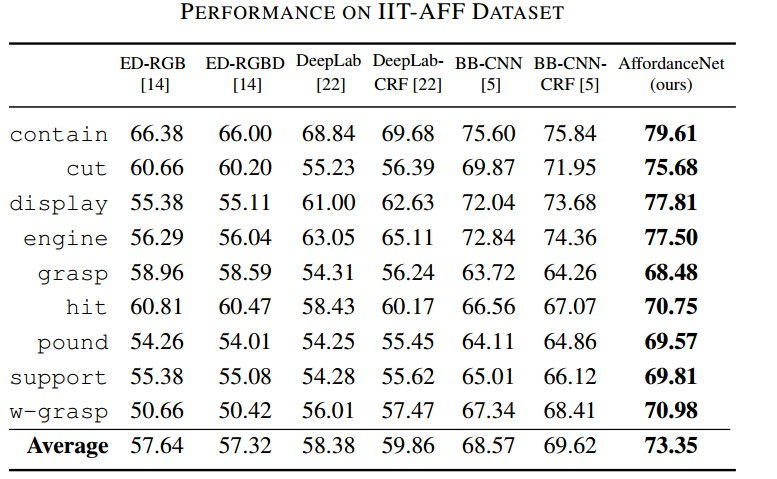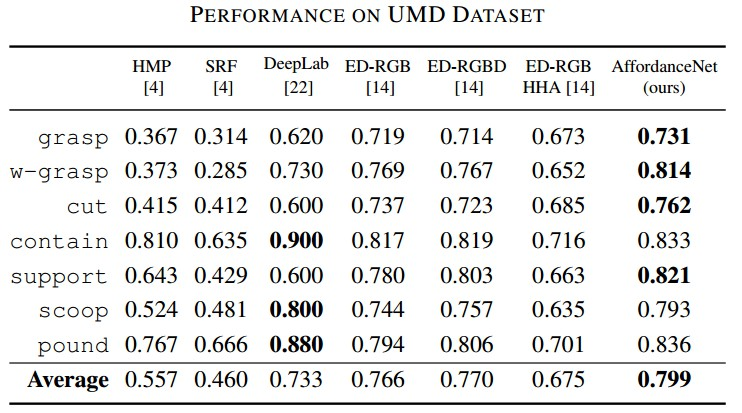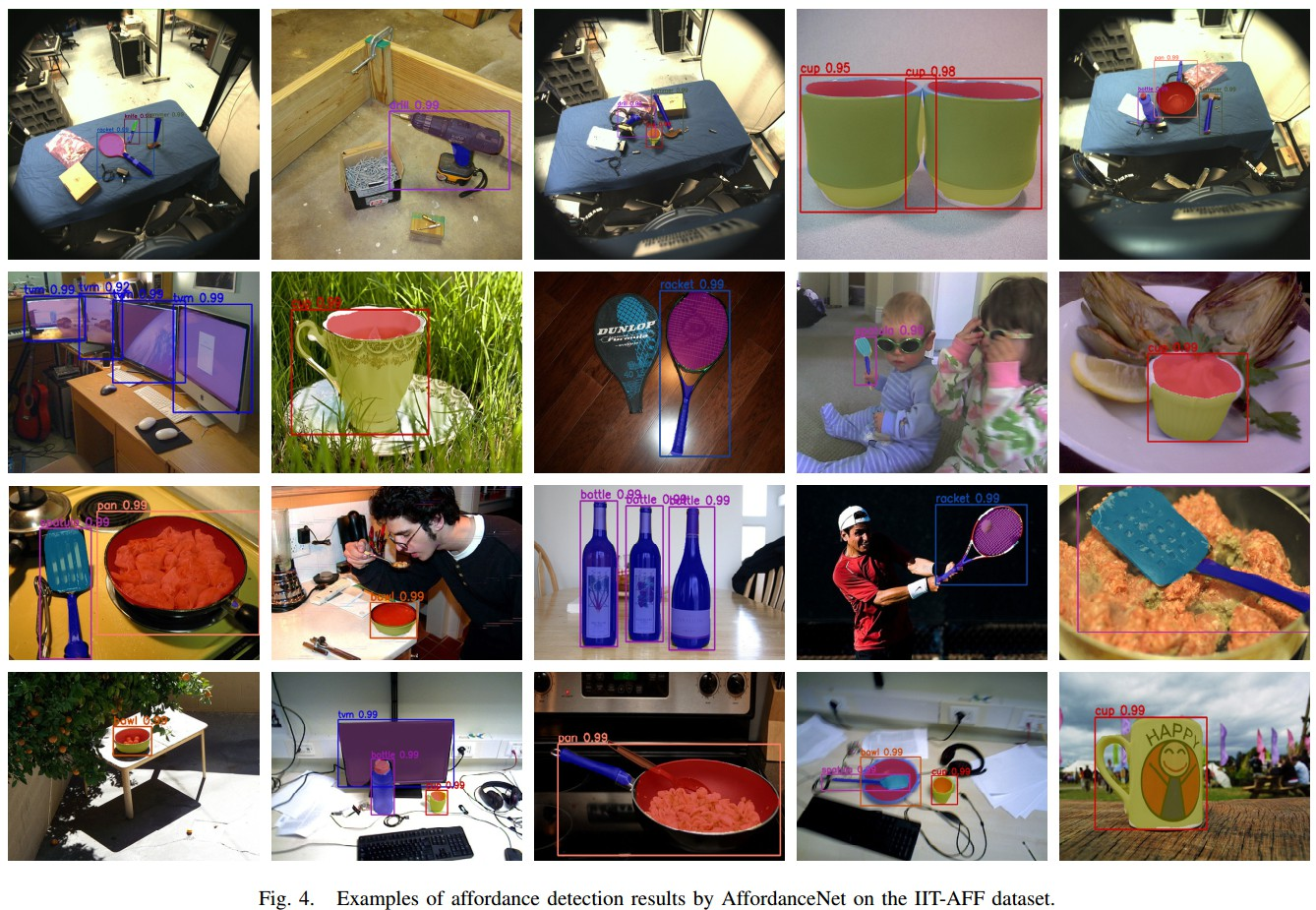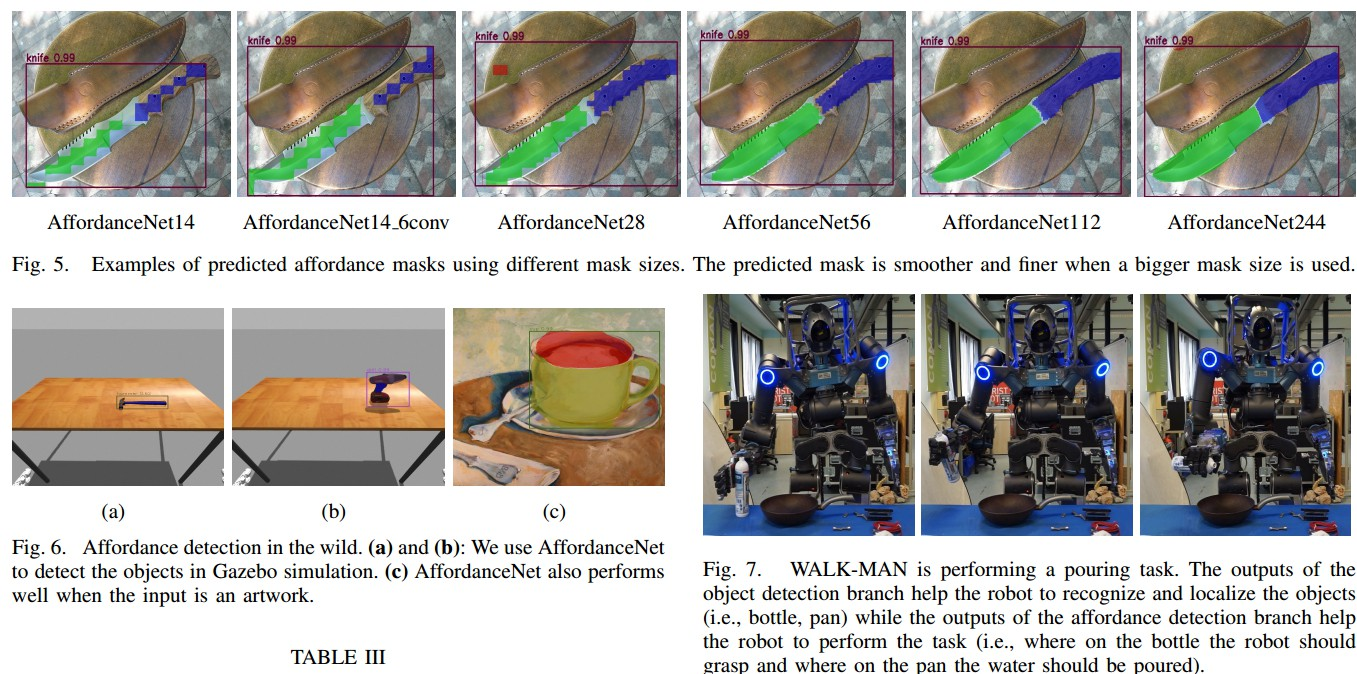Last modification：October 10th, 2018 at 12:29 am